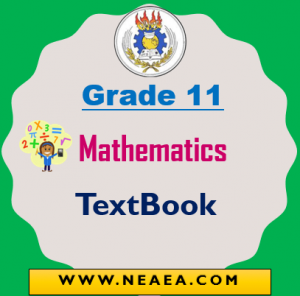However, the Ethiopian Ministry of education provides the Grade 11 Mathematics Textbook for students. Students can download the Grade 11 Mathematics in a PDF file for academic study purposes.In Grades 11 Mathematics courses will be different for natural science and social science stream students. Mathematics at these grade levels should be used as an important instrument for recognising and describing certain fields of objective reality as well as planning and guiding process of development.

Different topics from the history of Mathematics, facts and problems from social practice and other disciplines of science should be discussed and dealt with.

1 Unit: Further on Relation and Functions

• Further on Relation and Functions
• Revision on relations
• Some additional types of functions
• Classification of functions.
• Composition of functions
• Inverse functions and their graphs.

2 Unit: Rational Expressions and Rational Functions.

• Simplification of rational expressions
• Rational equations
• Rational functions and their graphs.

3 Unit: Coordinate Geometry

• Straight line
• Conic sections

4 Unit: Mathematical Reasoning

• Logic
• Arguments and validity

5 Unit: Statistics and Probability

• Statistics
• Probability

6 Unit: Matrices and Determinants

• Matrices
• Determinants and their properties
• Inverse of a square matrix
• Systems of equations with two or three variables
• Cramer’s rule

7 Unit: The Set of Complex Numbers

• The concept of complex numbers
• Operations on complex numbers
• Complex conjugate and modulus
• Simplification of complex numbers
• Argand diagram and polar representation of

8 Unit: Vectors and Transformation of the Plane (For Natural Science Students)

• Representation of vectors.
• Scalar (inner or dot) product of vectors
• Application of vector
• Transformation of the plane

9 Unit: Further on Trigonometric Functions  (For Natural Science Students)

• The functions y -sec x, y z csc x and y z cot x
• Inverse of trigonometric functions
• Graphs of some trigonometric functions
• Applications of trigonometric functions

10. Mathematical Applications in Business (For Social Science Students)

• Basic mathematical concepts in business.
• Compound interest and depreciation
• Saving, investing and borrowing money
• Taxation